Physics Department                [Return to Examples]

# Thermo Example 3© 2000 D.P. Maloney

Thirty liters of a monatomic ideal gas are confined in a cylinder with a moveable piston.  The gas starts out at a temperature of 37oC under a pressure of 1.5 atm.  We will call this condition of the gas State I.

(a)     How many moles of gas are present?

(b)     The gas is heated at constant pressure to twice the initial volume.  What is the temperature of the gas at the end of this process?

(c)     Starting with the gas in State I again, it undergoes an isothermal expansion to 60 l, what is the new pressure of the gas?

(d)     Again, the gas starts from State I.  This time it undergoes an adiabatic expansion to 60 l.  What are the temperature and pressure of the gas at the end of this process?

(e)     Finally, starting from State I, the gas undergoes a constant volume process to a pressure of 3 atm.  What is the final temperature of the gas at the end of this process?

Given: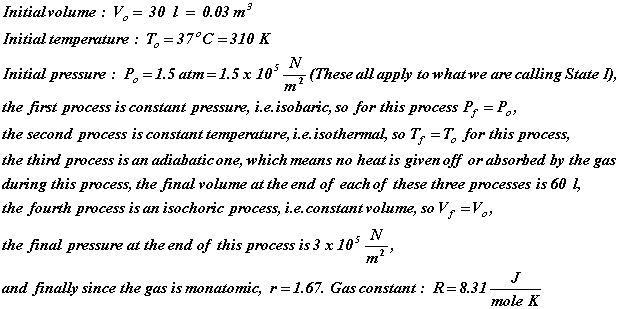Unknown: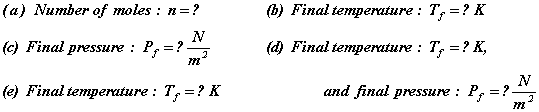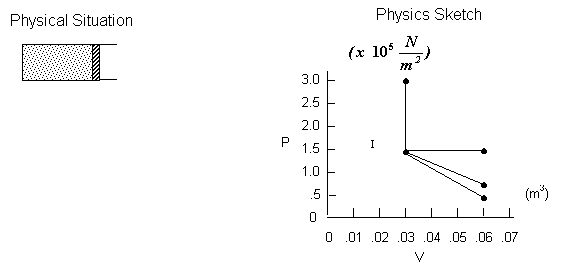Physical Principles and/or Ideas:    Behavior and relations for an ideal gas undergoing an isobaric, isothermal, isochoric and adiabatic process, and Ideal Gas Law.

Solution:         We will tackle the parts of this problem in sequence.  For part (a), we know the temperature, pressure and volume of the gas in State I.  We also know it is an ideal gas, so we can use the Ideal Gas Law and solve directly for the number of moles.  That is: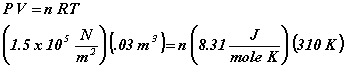Solving for n, we get: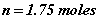In part (b), we are looking for the final temperature at the end of the isobaric process.  Since the pressure is a constant for this process, we can rearrange the Ideal Gas Law putting the variables on one side of the equality and the constants on the other side, that is: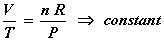This same constant applies to all states during the isobaric process, so: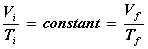Or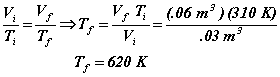The procedure for part (c) is similar to what we just did in part (b), but this time we have: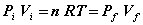So the pressure at the end of the isothermal process is: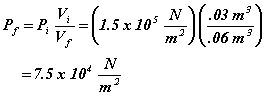The process in part (d) is an adiabatic one.  In such a process, there is no heat given off or absorbed by the gas.  In adiabatic processes, the temperature, pressure and volume of the gas all change, so we cannot use the Ideal Gas Law as we did in parts (b) and (c).  We have different relations for adiabatic processes.  They are: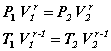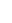where g = ratio of the specific heat at constant pressure to the specific heat at constant volume.  This ratio is a constant determined by the number of atoms in the molecule of the gas.  For a monatomic gas, its value is 1.67.We use the second of these two relations to find the temperature at the end of the adiabatic process.  Rearranging, we have: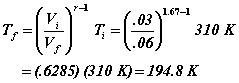We find the final pressure from the first relation above.  We get: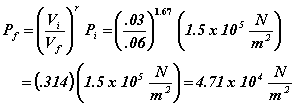It is always a good idea to check our work, so our first question about the last two values we have calculated is:  are they reasonable?  To answer this, we should think about what happens when a gas expands; its temperature and pressure both decrease.  The temperature and pressure values we calculated are both less than the values for State I, so our answers look reasonable from that perspective.  But can we do any more specific checking here?  The answer is yes because we are dealing with an ideal gas so the Ideal Gas Law must be satisfied at all times.  Plugging the values we calculated, and the final volume, into the Ideal Gas Law, we can solve for the number of moles of gas.  If the answer we get is the same as we found in part (a), we should have the correct final temperature and pressure.  Carrying out this calculation, we have: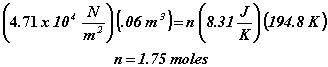So, we have reasonable assurance that our values are the appropriate ones.

The final process in this problem is an isochoric one to twice the pressure of State I.  To find the final temperature at the end of this process, we can again use the Ideal Gas Law.  Since volume is constant in this process, we have: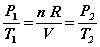So,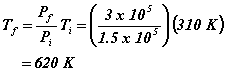Notice that this is the same final temperature as was found at the end of the isobaric process.

Other Thermo Examples:    1     2     3     4     5
[Examples Homepage]      [Physics Homepage]      [IPFW Homepage]